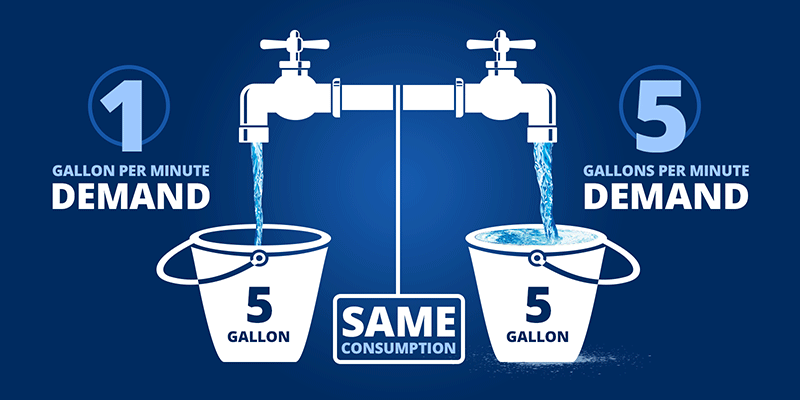# Time of day rates - understanding demand

An electric meter measures electricity in two ways:

1. kWh = kilowatt hours, which is a measure of consumption
2. kW = kilowatts in 15-minute intervals, which is a measure of demand

A demand measure represents the amount of power the utility must generate to fulfill the customer’s power needs.

#### Kilowatt vs. Kilowatt hour

One 100-watt light bulb burning for 10 hours consumes 1,000 watt-hours or 1 kWh. The entire time the light bulb is on, it requires or “demands” 100 watts or 0.1 kW from the utility.Note: In both examples, the consumption is 1 kWh, however, look how differently the second situation impacts the utility from a demand perspective. The serving utility must now be prepared to provide 10 times as much “capacity” in response to the “demand” of the 10 light bulbs operating all at once.

This means the utility must have 0.1 kW ready whenever a customer turns on a light.

Similarly, ten 100-watt light bulbs burning for one hour consume 1,000 watt-hours or 1kWh.

A different way to look at demand and consumption is with the scenario of filling a 5 gallon bucket with water.Note: The low flow faucet fills the bucket slower than the high flow faucet. The flow rate is the equivalent to demand, and the 5 gallons of water are equivalent to consumption.  In this example, filling both buckets has the same "consumption" but very different "demands."